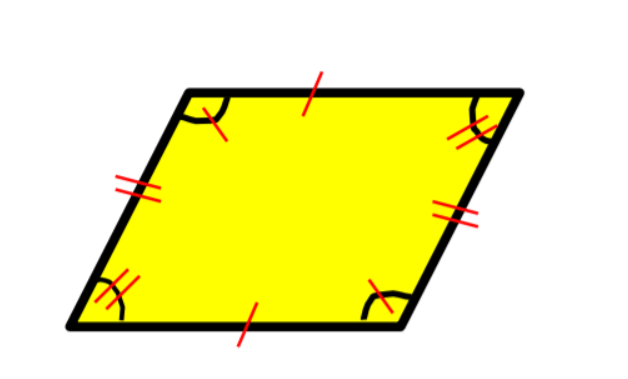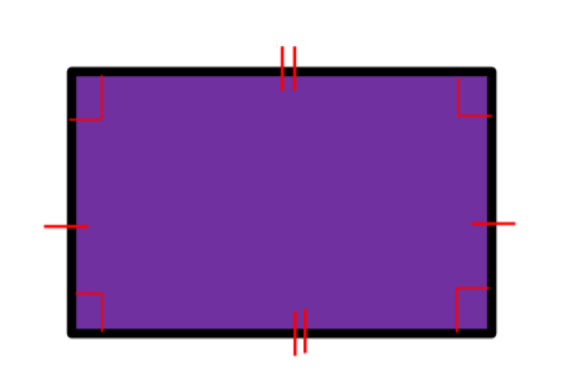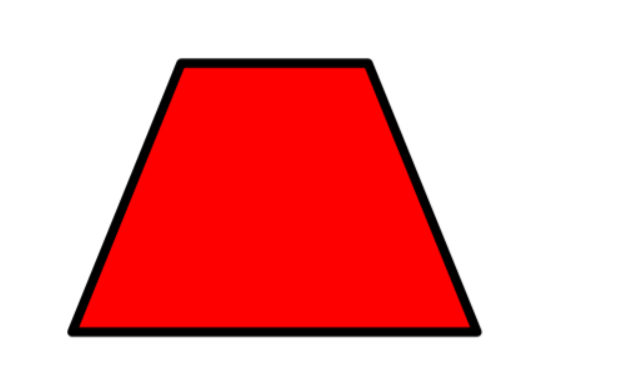Listen to this Lesson:

In the fifth grade, students learn what quadrilaterals are, get familiarized with the different types of quadrilaterals, and become fluent in determining the properties of each quadrilateral. They also learn how to draw quadrilaterals.

There are many ways in which math teachers can make lessons on quadrilaterals more engaging. To help out, we’ve included awesome teaching tips and games that blend learning with fun when explaining ‘what are quadrilaterals’ to your students!

You can start your lesson on quadrilaterals by defining them. Point out that a quadrilateral is a 2-dimensional figure because it has a flat shape. Add that the word ‘quad’ means ‘four’ and ‘lateral’ means ‘sides’. Thus, a quadrilateral is any closed figure that has ‘four sides’.

Add that there are different types of quadrilaterals and draw one type as an example:Explain that there are certain characteristics, or properties, that all quadrilaterals share, regardless of the type of quadrilateral. You can enumerate these properties in the following way on the whiteboard:

• a quadrilateral has four vertices
• a quadrilateral has four sides
• a quadrilateral has four angles
• a quadrilateral has 2 diagonals

Draw a quadrilateral on the whiteboard and ask students to identify each property. What are the four vertices, sides, and angles of the given quadrilateral? What are its two diagonals?### Types of Quadrilaterals and their Properties

As mentioned earlier, there are several types of quadrilaterals. Explain each quadrilateral and its distinct properties and provide a drawing on the whiteboard.

#### Parallelogram

Point out that a parallelogram is a quadrilateral that has 4 sides, 2 pairs of opposite sides that are parallel, 2 pairs of opposite sides that are equal, as well as 2 pairs of opposite angles that are equal. Provide an example:#### Rhombus

Explain that a rhombus is a quadrilateral that has 4 equal sides, 2 pairs of parallel sides, and 2 pairs of opposite angles that are equal. Provide an example:#### Rectangle

Explain that a rectangle is a quadrilateral that has 2 pairs of opposite sides that are congruent, 2 pairs of parallel sides, and 4 right angles. Provide an example:#### Square

Explain that a square is a quadrilateral that has 4 equal sides, 2 pairs of parallel sides, as well as 4 right angles. Provide an example:#### Trapezoid

Explain that a trapezoid is a quadrilateral that has 4 sides and one pair of parallel sides. It can have a pair of equal sides, a pair of equal angles, and right angles. Provide an example:### Blue & Red Hearts Game

In this fun game, students will practice applying their knowledge of the different types of quadrilaterals, as well as determining the properties of each quadrilateral. To use this activity in your class, you’ll need to print out page 1 of this Assignment Worksheet (Members Only).

Divide students into groups of 3 or 4 and hand out the copies. The worksheet contains several statements on quadrilaterals, with a heart underneath. If the statement is correct, students should color the heart blue, and if it’s incorrect, they should color it red.

Students in each group work together to finish coloring all the hearts. Once students complete the activity, create space for discussion and reflection. Were some statements more challenging than others? Can students explain why they colored some statements red and others blue?In this interactive online game, students get to practice classifying quadrilaterals, by relying on what they’ve learned so far on the types of quadrilaterals and their properties. To implement this game in your class, make sure there are enough devices for all students.

Students play the game in pairs. In each exercise, they have to classify the given image of a quadrilateral, based on its properties, and select all the answers that apply. If they get stuck, they can also choose to use a hint for help.

Once they’ve completed the exercises, students in each pair compare their scores. The person with the highest score wins the game. Homeschooling parents can also use the game as individual practice.

## Before You Leave…

If you enjoyed these ideas and activities for teaching ‘What are quadrilaterals?’ to your student, you’ll want to check out our lesson that’s dedicated to this topic! So if you need guidance to structure your class and teach it, sign up for our emails to receive loads of free content!

Feel free to also check out our blog – you’ll find plenty of awesome resources that you can use in your class, such as this awesome article with free worksheets and activities on classifying 2-dimensional shapes!